# 【数学建模】二 ：MATLAB 数学建模快速入门

MATLAB 虽然功能很强大，但上手却很容易，尤其是 R2013a 以后的版本， 使用起来更加容易。 现在使用 MATLAB 的一个理念就是像 Word 一样去使用 MATLAB。本讲将通过一个实例介绍如何像使用 Word 一样去使用 MATLAB，真正将 MATLAB 当工具来使用，即使对于从来没有用过 MATLAB 的模友来说，也可以轻松入门。

1. MATLAB 的功能1. 快速入门案例

MATLAB 虽然是一款程序开发工具，但依然是工具，所以它可以像其他工具（如 Word）一样易用。而传统的学习 MATLAB 方式一般是从学习 MATLAB 入门知识开始，比如 MATLAB 矩阵操作、绘图、数据类型、程序结构、数值计算等内容。学这些知识的目地是能够将 MATLAB 用起来，可是即便学完了，很多人还是不自信自己能独立、自如地使用 MATLAB。这是因为在我们学习这些知识的时候，目标是虚无的，不是具体的目标，具体的目标应该是要解决某一问题。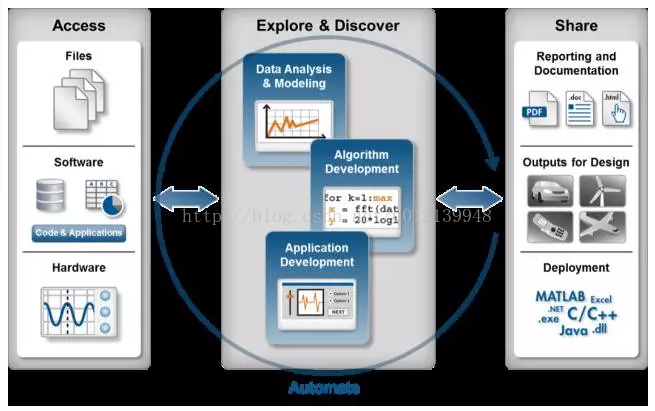Step1.1：选中数据文件，右键，将弹出右键列表，很快可发现有个“导入数据”菜单，如图 3 所示。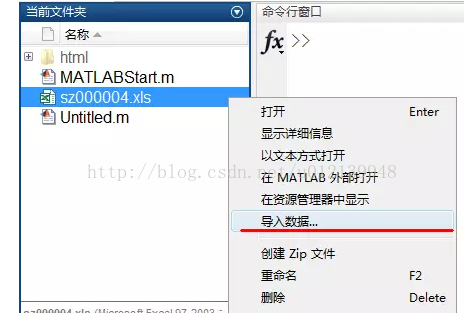Step1.2：单击“导入数据”这个按钮，则很快发现起到一个导入数据引擎，如图 4 所示。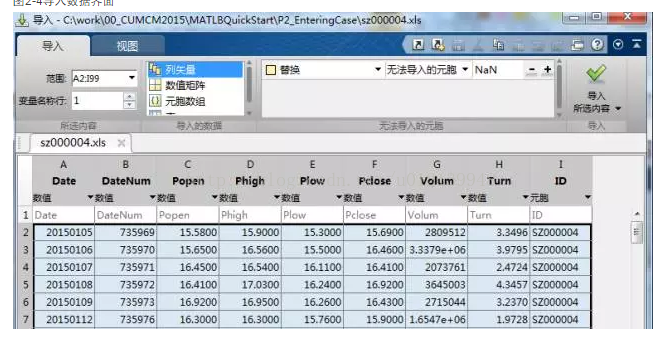图4 导入数据界面

Step1.3：观察图 4，只在右上角有个“导入所选内容”按钮，则可直接单击之。马上我们就会发现在 MATLAB 的工作区（当前内存中的变量）就会显示这些导入的数据，并以列向量的方式表示，因为默认的数据类型就是“列向量”，当然您可以可以选择其他的数据类型，大家不妨做几个实验，观察一下选择不同的数据类型后会结果会有什么不同。至此，第一步获取数据的工作的完成。

Step2.1：查看数据的统计信息，了解我们的数据。具体操作方式是双击工具区（直接双击这三个字），此时会得到所有变量的详细统计信息。通过查看这些基本的统计信息，有助于快速在第一层面认识我们所正在研究的数据。当然，只要大体浏览即可，除非这些统计信息对某个问题都有很重要的意义。数据的统计信息是认识数据的基础，但不够直观，更直观也更容易发现数据规律的方式就是数据可视化，也就是以图的形式呈现数据的信息。下面我们将尝试用 MATLAB 对这些数据进行可视化。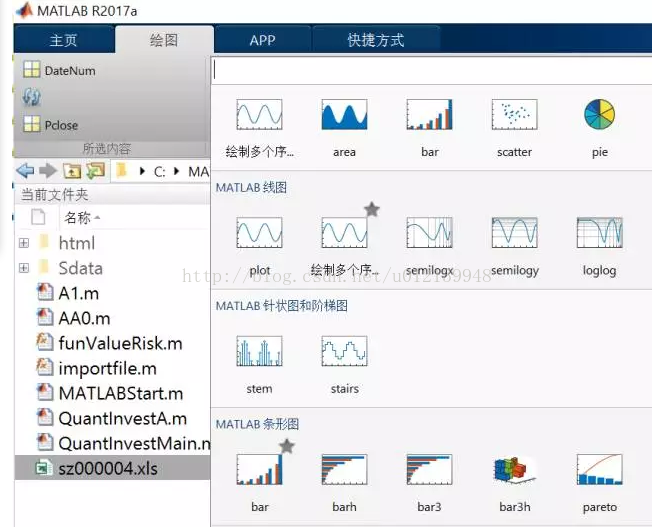Step2.2：选中变量 DataNum 和 Pclose，在绘图面板中单机 plot 图标，马上可以得到这两个变量的可视化结果，如图 6 所示，同时还可以在命令窗口区显示绘制此图的命令：

plot(DateNum,Pclose)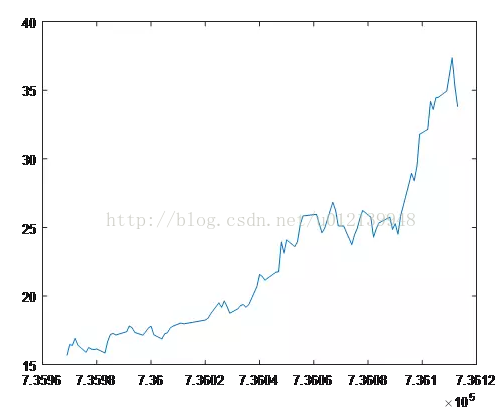（1）曲线的颜色、线宽、形状；

（2）坐标轴的线宽、坐标，增加坐标轴描述；

（3）在同个坐标轴中绘制多条曲线。

Step2.3：通过帮助搜索多项式拟合的命令，并计算股票的价值，具体代码为：

p = polyfit(DateNum,Pclose,1); % 多项式拟合

value = p(1) % 将斜率赋值给value，作为股票的价值

value =

0.1212


Step2.4：用相似的方法，可以很快得到计算最大回撤的代码：

MaxDD = maxdrawdown(Pclose); % 计算最大回撤

risk = MaxDD % 将最大回撤赋值给risk，作为股票的风险

risk =

0.1155


Step2.5：像 Step1.1 一样，重新选中数据文件，右键并单击“导入数据”菜单，待启动导入数据引擎后，选择“生成脚本”，然后就会得到导入数据的脚本，并保存该脚本。

Step2.6：从命令历史中选择一些有用的命令，并复制到 Step2.5 得到的脚本中，这样就很容易得到了解决该问题的完整脚本了，如下所示：

%% MATLAB 入门案例

%% 导入数据

clc, clear, close all

% 导入数据

% 创建输出变量

data = reshape([raw{:}],size(raw));

% 将导入的数组分配给列变量名称

Date = data(:,1);

DateNum = data(:,2);

Popen = data(:,3);

Phigh = data(:,4);

Plow = data(:,5);

Pclose = data(:,6);

Volum = data(:,7);

Turn = data(:,8);

% 清除临时变量

clearvars data raw;

%% 数据探索

figure % 创建一个新的图像窗口

plot(DateNum,Pclose,’k’) % 更改图的的颜色的黑色（打印后不失真）

datetick(’x’,‘mm’);% 更白日期显示类型

xlabel(’日期‘); % x轴说明

ylabel(’收盘价‘); % y轴说明

figure

bar(Pclose) % 作为对照图形

%% 股票价值的评估

p = polyfit(DateNum,Pclose,1); % 多项式拟合,

% 分号作用为不在命令窗口显示执行结果

P1 = polyval(p,DateNum); % 得到多项式模型的结果

figure

plot(DateNum,P1,DateNum,Pclose,’*g’); % 模型与原始数据的对照

value = p(1) % 将斜率赋值给value，作为股票的价值。

%% 股票风险的评估

MaxDD = maxdrawdown(Pclose); % 计算最大回撤

risk = MaxDD % 将最大回撤赋值给risk，作为股票的风险

Step3.1：在脚本编辑器的“发布”面板，从“发布”按钮（最右侧）的下拉菜单中，选择“编辑发布选项”，这样就打开了发布的配置面板。

Step3.2：根据自己的要求，选择合适的“输出文件格式”，默认为 html，但比较常用的是 Word 格式，因为 Word 格式便于编辑，尤其是对于写报告或论文。然后单击“发布”按钮，就可以运行程序，同时会得到一份详细的运行报告，包括目录、实现过程、主要结果和图，当时也可以配置其他选项来控制是否显示代码等内容。

1. 入门后的提高©️2019 CSDN 皮肤主题: 大白 设计师: CSDN官方博客